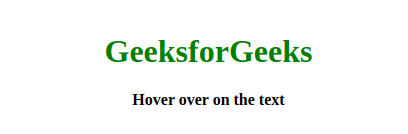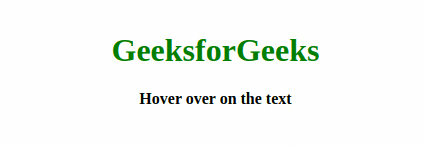Related Articles
Rubber Band Text animation using HTML and CSS
• Last Updated : 22 May, 2020The rubber band effect is quite popular and it looks attractive when a text string becomes stretchable obviously that will look cool. The rubber band text animation can be easily generated using CSS animations, we will use the @keyframes rule to get the desired output. So we will divide the article into two sections in the first section we will create the text string which will be rubbery when the user hover over on the text string. That effect we will add in the second section.

Creating Structure: In this section we will just use the HTML to create the text string.

• HTML Code: In this section we will create a basic text using the h1 tag.
 ```<``html` `lang``=``"en"` `dir``=``"ltr"``>``    ``<``head``>``        ``<``meta` `charset``=``"utf-8"` `/>``        ``<``title``>Rubber Band Text Animation``         ` `    ````    ``<``body``>``        ``<``div` `id``=``"text"``>``            ``<``h1``>GeeksforGeeks``            ``<``b``>Hover over on the text``        ````    `````

Designing Structure: In this section we will design the structure to make the created text string rubbery.

• CSS Code: In this section we will create the animation effect using CSS animations, we will use the @keyframes rule to specify the animation code. We will use the tranform property to produce the animation
 ``

Final Solution: It is the combination of the above two code sections.

 ```<``html` `lang``=``"en"` `dir``=``"ltr"``>``    ``<``head``>``        ``<``meta` `charset``=``"utf-8"` `/>``        ``<``title``>Rubber Band Text Animation``        ``<``style``>``            ``body {``                ``margin: 0;``                ``padding: 0;``                ``font-family: serif;``            ``}`` ` `            ``#text {``                ``position: relative;``                ``margin-top: 100px;``                ``text-align: center;``            ``}`` ` `            ``h1 {``                ``color: green;``            ``}`` ` `            ``#text:hover {``                ``animation: effect linear 1s;``            ``}`` ` `            ``@keyframes effect {``                ``0% {``                    ``transform: scale(1, 1);``                ``}``                ``25% {``                    ``transform: scale(1.3, 0.6);``                ``}`` ` `                ``50% {``                    ``transform: scale(1.1, 0.9);``                ``}``                ``100% {``                    ``transform: scale(1, 1);``                ``}``            ``}``        ````    ````    ``<``body``>``        ``<``div` `id``=``"text"``>``            ``<``h1``>GeeksforGeeks``            ``<``b``>Hover over on the text``        ````    `````

Output:My Personal Notes arrow_drop_up